Bessel processes

A family of continuous Markov processes (cf. Markov process)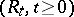taking values in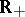, parametrized by their dimension.

Whenis an integer,may be represented as the Euclidean norm of Brownian motion in. Letbe the law of the square, starting from, of such a process, considered as a random variable taking values in. This law is infinitely divisible (cf. [a6] and Infinitely-divisible distribution). Hence, there exists a unique familyof laws onsuch that(a1)

(indicates the convolution of probabilities on), which coincides with the family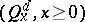, for integer dimensions.

The process of coordinateson, under, satisfies the equation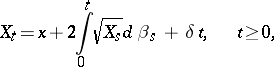(a2)

witha one-dimensional Brownian motion. Equation (a2) admits a unique strong solution, with values in. Call its square root a-dimensional Bessel process.

Bessel processes also appear naturally in the Lamperti representation of the process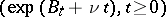, where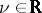and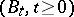denotes a one-dimensional Brownian motion. This representation is: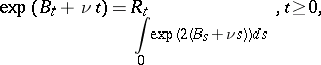(a3)

whereis a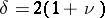-dimensional Bessel process. This representation (a3) has a number of consequences, among which absolute continuity properties of the laws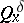asvaries and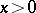is fixed, and also the fact that a power of a Bessel process is another Bessel process, up to a time-change.

Special representations of Bessel processes of dimensions one and three, respectively, have been obtained by P. Lévy, as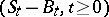, and by J. Pitman as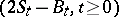, where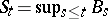, andis a one-dimensional Brownian motion.

Finally, the laws of the local times ofconsidered up to first hitting times, or inverse local times, can be expressed in terms ofand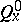, respectively: this is the content of the celebrated Ray–Knight theorems (1963; [a1], [a5]) on Brownian local times. These theorems have been extended to a large class of processes, including real-valued diffusions.